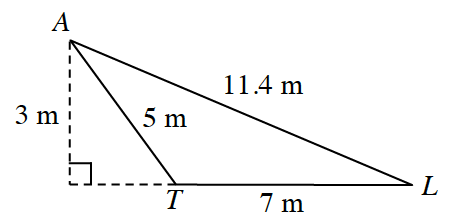### Home > INT2 > Chapter 6 > Lesson 6.2.3 > Problem6-76

6-76.Al notices that the ratio of the perimeters of two similar polygons is equal to the ratio of their side lengths. “What about their areas? Are they related in the same way?” he wonders.

1. What are the perimeter and area of $∆ALT$?

$P = 7 + 5 + 11.4 m$
$A = (1/2)(7)(3) m^2$

2. Test Al’s question by enlarging $∆ALT$ by a scale factor of $3$. What are the perimeter and area of the image triangle?

Use the new side lengths to compute the area and perimeter of the enlarged triangle.

3. Does the perimeter increase by a scale factor of $3$?

$\frac{70.2}{23.4} = ?$

Yes!

4. Answer Al’s question. Does the area increase by a scale factor of $3$? Explain what happened.

$\frac{94.5}{10.5} = ?$